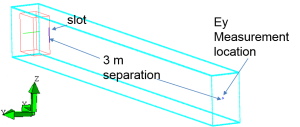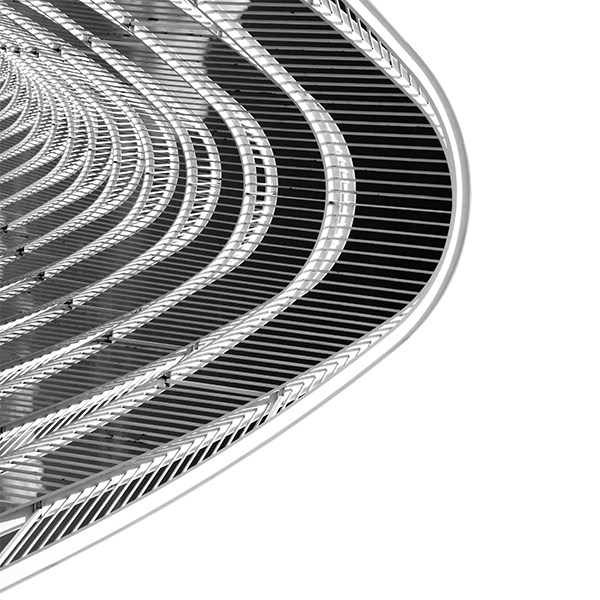# EMI from a Slot in a Box

## Benchmark Simulation Using EMA3D

It is important to demonstrate that EM simulation packages can reproduce measured data for problems of interest. EMI from a slot is a common EMC concern. This demonstration compares an EMA3D simulation to an existing measurement in the literature.

The electromagnetic interference (EMI) from a slot in a rectangular box has been explored using a FDTD technique and compared to measurements. An FDTD simulation software, EMA3D,  has been used to analyze a similar problem and the results are presented herein. The simulation files, results and plotting scripts have been provided to assure the validity of the EMA3D modeling results.

## EMA3D Model Setup

Mesh size: Δx = 1 cm, Δy = 0.5 cm, Δz = 1 cm

A thin wire with termination impedances of R1 = 50 Ω, and R2 = 47 Ω was used to model the source line (green line). A thin gap formalism was used to model the slot (blue line) with a width of 1 mm. Box surfaces (Lx = 22 cm, Ly = 14 cm, Lz = 30 cm) were modeled as perfect electrical conductors (PEC). A Gaussian pulse voltage source with amplitude 1 mV was used at the R1 end of the thin wire.## EMA3D Simulation Setup

A far-field electric field approximation was used to determine the electric field at a 3 m distance from the slot in the benchmark. EMA3D has the ability to obtain far-field approximations similar to the ones performed in the paper. Because the problem is relatively small for the computing resources available, the actual electric field was computed at the 3 m distance. Only the y component of the electric field was considered.## EMA3D Results Vs. Results in Benchmark 

The plots demonstrate that the EMA3D simulation results compare well to the benchmark results. Every cavity-mode and slot generated resonance produced in the benchmark problem is captured in the EMA3D results for delivered power and electric field at 3 m.

## Instruction Document to Reproduce the Results

Slotted_Box_Instructions

1.M. Li, J. Nuebel, J. L. Drewniak, R. E. DuBroff, T. H. Hubing, T. P. Van Doren, “EMI from Cavity Modes of Shielding Enclosures – FDTD Modeling and Measurements,”  IEEE Transactions on Electromagnetic Compatibility, Vol. 42, No. 1, pp. 29 – 38, Feb. 2000